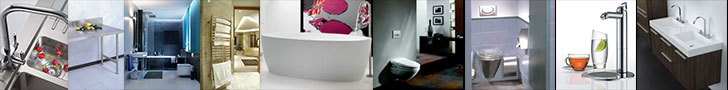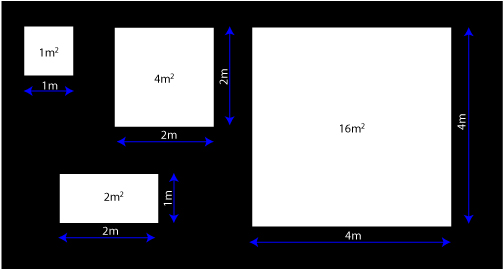Sales/Quotes:   012 809 4026   antonius@chilli-b.co.za   retha@chilli-b.co.zaSo many people, even tilers and builders, battle with this calculation. We even have architects phoning us to do the calculation. First of all we need to know what is a square meter. Well, it is an area which is 1 m wide by 1 m long. About the size of an average shower (which is usually 0.9 m wide and 0.9 m long. So it is a square. If the area is 2 m long and 0.5 m wide then it is also 1 square meter, i.e. 1 m² , although the shape is rectangular.Increasing the length and width quadruples the surface area to be tiled. The surface area is obtained by multiplying the length and width of the area

So lets take an example. You have a shower floor which is 0.9 m wide (or 900 mm) and 0.9 m long (or 900 mm). So the calculated total surface area is 0.9 x 0.9 = 0.81 m². Let us assume you would like to install a mosaic on the floor of the shower. The size of the mosaic is 300 mm wide by 300 mm long (or wide), which is also a square (it usually is with mosaics). So the calculated total surface area of a mosaic is 0.3 x 0.3 = 0.09 m². As you can see the value is smaller than the 0.81 m² required for the shower floor. In order to calculate the amount of mosaic sheets required to cover the shower floor we use the following formula:$\large\textit{Number of mosaic sheets}=\dfrac{\large\text{shower width} \times \large\text{shower length}}{\large\text{mosaic width}\times\large\text{mosaic length}}$$\large\textit{Number of mosaic sheets}=\dfrac{\large\text{0.9} \times \large\text{0.9}}{\large\text{0.3}\times\large\text{0.3}}$$\large\textit{Number of mosaic sheets}=\dfrac{0.81} {0.09}=9$

So we need 9 sheets to cover the complete shower floor with a mosaic sheet size of 300 x 300 mm. Please always order more than you need. There are obstacles such as the shower drain. This can cause a lot of problems for tillers since they need to cut a hole in the mosaic sheet. Quite often they bugger up this process and ruin a complete mosaic sheet, so you must buy extra. The formula is usually 5-10% extra. In the case of a small area and large mosaic sheets, one should suffice, but you never know.

Let us use another sample. To cover your wall behind the kitchen stove with metro tiles. Just like a glass kitchen splashback. First, measure the length and width of the area that must be covered. You do not have to be too exact, use your kids ruler, the 300 mm one (not 150 mm, too much effort). Ok, so now measure the width. It should be about 800-900 mm for an average stove width. Now measure the height. Take it from the work surface top to just under the extractor fan, that should be about 800 – 900 mm. Lets take it you measured 865 mm x 925 mm. That is 0.865 m x 0.925 m = 0.8 m². The metro tiles are 75 mm x 150 mm. So the formula is as follows:$\large\textit{Number of metro tiles}=\dfrac{\large\text{stove wall width} \times \large\text{stove wall length}}{\large\text{metro tile width}\times\large\text{metro tile length}}$$\large\textit{Number of mosaic sheets}=\dfrac{{0.865} \times {0.925}}{{0.075}\times {0.15}}$

Remember 75 mm = 0.075 m and 150 mm = 0.15 m. We always work in meter (m) and not in milli-meters (mm) since the numbers just get astronomic, in any way, a retailer will faint if you tell him you need 800,125.00 mm² of metro tiles….I will anyway.$\large\textit{Number of metro tiles}=\dfrac{0.8} {0.01}=80$

Remember to add the 5-10 % for breakage. So you need 80 metro tiles plus 80 + 5% = 84 metro tiles at least. I would order 88, which allows for 10 % breakage, but that is me and I am selling the stuff.